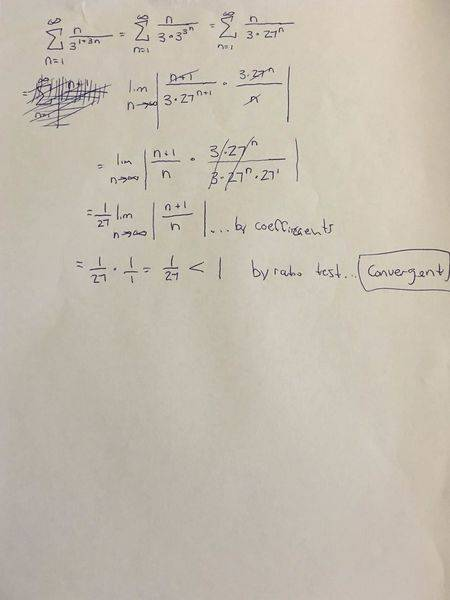# Question over a Series

## Homework Statement

I was watching a PatrickJMT video on ratio test and he gave this problem. I solved it before he did, and he got that it was divergent. He didn't simplify it initially, so our methods of approach are different. Did I do something wrong? I checked my calculator to make sure all my simplifications were correct. So I'm assuming I did something near the limit portion that was incorrect (if at all). Thanks

∑ n/31+3n
n=1

## The Attempt at a Solution

∑ n/31+3n = n/(3⋅27n)
n=1

lim |(n+1)/(3⋅27n+1) / (n/3 *27n)|
n→∞

= lim |(n+1)/n| * (1/27)
n→∞
= 1 * 1/27
=1/27 < 1

Convergent by Ratio Test

member 587159
Did you use the ratio test correctly?Did you use the ratio test correctly?
Nope. Got it, thanks.

Actually I typed my work incorrectly. I'll fix it. but to be sure...

Ray Vickson
Homework Helper
Dearly Missed

## Homework Statement

I was watching a PatrickJMT video on ratio test and he gave this problem. I solved it before he did, and he got that it was divergent. He didn't simplify it initially, so our methods of approach are different. Did I do something wrong? I checked my calculator to make sure all my simplifications were correct. So I'm assuming I did something near the limit portion that was incorrect (if at all). Thanks

∑ n/31+3n
n=1

## The Attempt at a Solution

∑ n/31+3n = n/(3⋅27n)
n=1

lim |(n+1)/(3⋅27n+1) / (n/3 *27n)|
n→∞

= lim |(n+1)/n| * (1/27)
n→∞
= 1 * 1/27
=1/27 < 1

Convergent by Ratio Test

It is convergent, as you have shown correctly using the ratio test.

You can actually find the sum ##\sum_{n=1}^N n/3^{3n+1}## explicitly (as a reasonably simple closed-from expression in ##N##), then take the limit as ##N \to \infty##. All you need to do is find a formula for ##f_N(x) =\sum_{n=1}^N n x^n##, and these are widely available, or you can work it out as ##f_N(x) = x (d/dx) g_N(x)##, where ##g_N(x) = \sum_{n=1}^N x^n##, which is elementary.

Last edited: